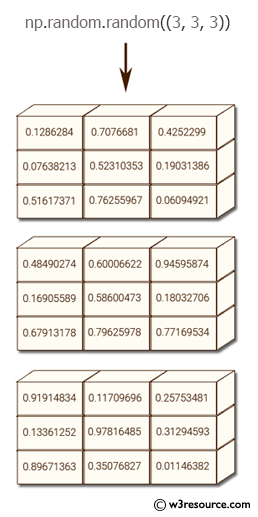﻿ NumPy: Create a 3x3x3 array filled with arbitrary values - w3resource# NumPy: Create a 3x3x3 array filled with arbitrary values

## NumPy: Basic Exercise-31 with Solution

Write a NumPy program to create a 3x3x3 array filled with arbitrary values.

Sample Solution :

Python Code :

``````import numpy as np
x = np.random.random((3, 3, 3))
print(x)
``````

Sample Output:

```[[[ 0.51919099  0.31268732  0.58506582]
[ 0.12730206  0.30556451  0.55981097]
[ 0.92910493  0.73947119  0.14252086]]

[[ 0.96159407  0.21341612  0.6814465 ]
[ 0.71884351  0.01271011  0.98812225]
[ 0.47553515  0.04584955  0.59425412]]

[[ 0.44064227  0.26612916  0.58861619]
[ 0.37935141  0.0640071   0.53717733]
[ 0.77986385  0.0771148   0.92183522]]]
```

Pictorial Presentation:Python Code Editor:

Have another way to solve this solution? Contribute your code (and comments) through Disqus.

What is the difficulty level of this exercise?

Test your Python skills with w3resource's quiz

﻿

## Python: Tips of the Day

Checks if the given number falls within the given range.

Example:

```def tips_range(n, start, end = 0):
return start <= n <= end if end >= start else end <= n <= start
print(tips_range(2, 4, 6))
print(tips_range(4, 8))
print(tips_range(1, 3, 5))
print(tips_range(1, 3))
```

Output:

```False
True
False
True
```